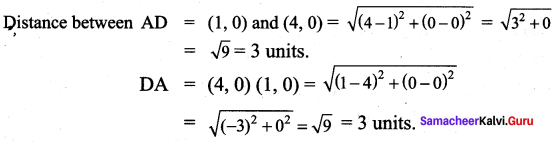## Tamilnadu Samacheer Kalvi 9th Maths Solutions Chapter 5 Coordinate Geometry Additional Questions

Exercise 5.1

Question 1.
State whether the following statements are true/false.
(i) (5, 7) is a point in the IV quadrant.
(ii) (-2, -7) is a point in the III quadrant.
(iii) (8, -7) lies below the x-axis.
(iv) (-2, 3) lies in the II quadrant.
(v) For any point on the x-axis its y-coordinate is zero.
Solution:
(i) False
(ii) True
(iii) True
(iv) True
(v) True

Question 2.
Locate the points
(i) (3, 5) and (5, 3)
(ii) (-2, -5) and (-5, -2) in the rectangular coordinate system.
Solution: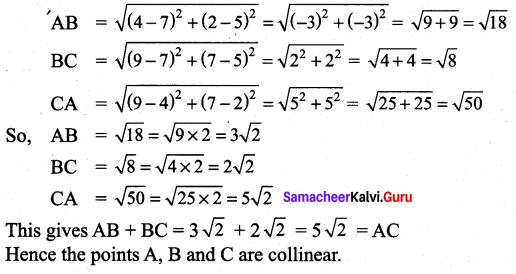Question 3.
In which quadrant does the following points lie?
(i) (5, 2)
(ii) (-5, -8)
(iii) (-7, 1)
(iv) (8, -3)
Solution:

Question 4.
Write down the ordinate of the following points.
(i) (7, 5)
(ii) (2, 9)
(iii) (-5, 8)
(iv) (7, -4)
Solution:
(i) 5
(ii) 9
(iii) 8
(iv) -4 (ordinate is the y-coordinate)

Exercise 5.2

Question 1.
Find the distance between the following pairs of points.
(i) (-4, 0) and (3, 0)
(ii) (-7, 2) and (5, 2)
Solution:
(i) The points (-4, 0) and (3, 0) lie on the x-axis. Hence,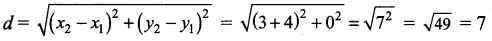(ii) The points (5,2) and (-7,2) lie on a line parallel to the x-axis. Hence the distance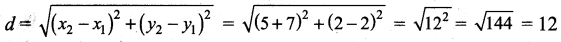Question 2.
Show that the three points (4, 2), (7, 5) and (9, 7) lie on a straight line.
Solution:
Let the points be A(4, 2), B(7, 5) and C(9, 7). By the distance formula.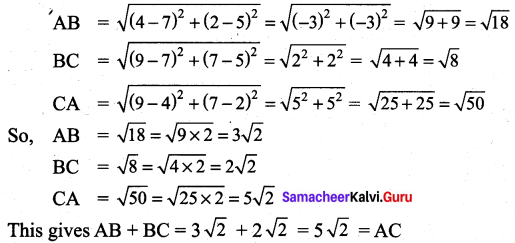Hence the points A, B and C are collinear.

Question 3.
Determine whether the points are vertices of a right triangle A(-3, -4), B(2, 6) and C (-6, 10).
Solution: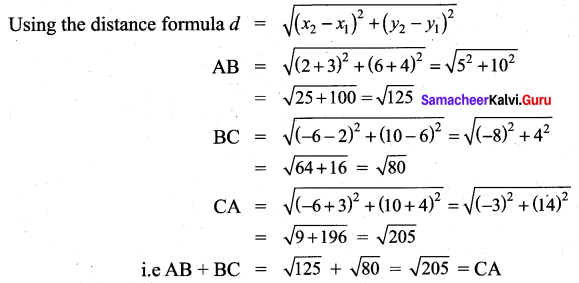Hence ABC is a right angled triangle since the square of one side is equal to sum of the squares of the other two sides.

Question 4.
Show that the points (a, a), (-a, -a) and ($$-a \sqrt{3}, a \sqrt{3}$$) form an equilateral triangle.
Solution:
Let the points be represented by A (a, a), B(-a, -a) and C($$-a \sqrt{3}, a \sqrt{3}$$) using the distance formula.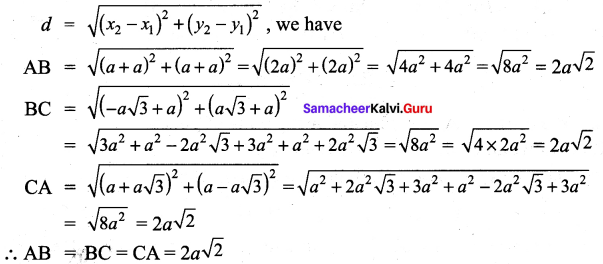Since all the sides are equal the points form an equilateral triangle.

Question 5.
Prove that the points (-7, -3), (5, 10), (15, 8) and (3, -5) taken in order are the corners of a parallelogram.
Solution:
Let A, B, C and D represent the points (-7, -3), (5, 10), (15, 8) and (3, -5) respectively.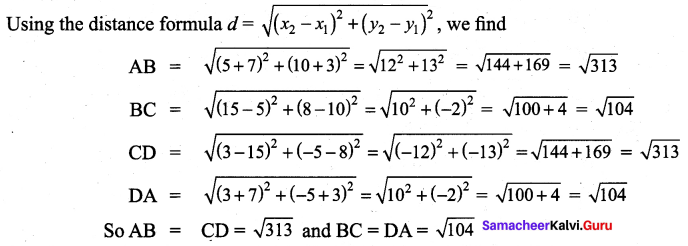i.e. The opposite sides are equal. Hence ABCD is a parallelogram.

Question 6.
Show that the following points A (3, 1) B(6, 4) and C(8, 6) lies on a straight line.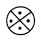Solution:
Using the distance formula, we have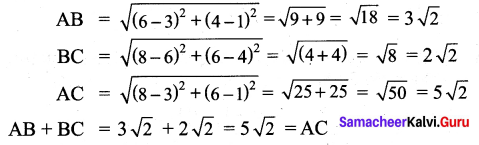Therefore the points lie on a straight line.

Question 7.
If the distance between the points (5, -2), (1, a) is 5 units. Find the value of a.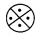Solution: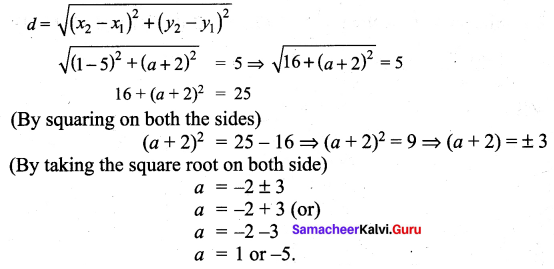Exercise 5.3

Question 1.
A, B and C are vertices of ∆ ABC. D, E and F are mid points of sides AB, BC and AC respectively. If the coordinates of A, D and F are (-3, 5), (5, 1) and (-5, -1) respectively. Find the coordinates of B, C and E.
Solution: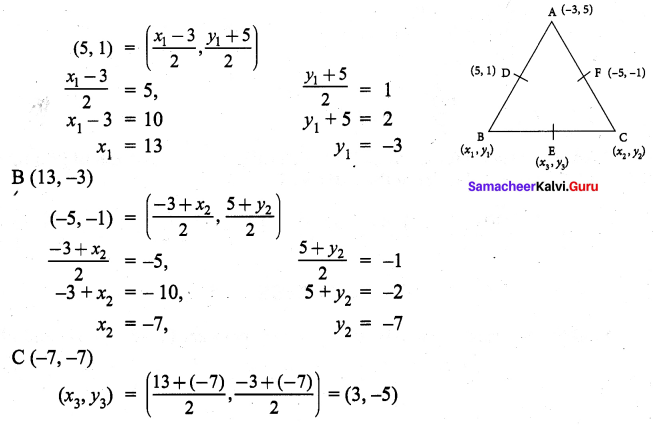Question 2.
If A(10, 11) and B(2, 3) are the coordinates of end points of diameter of circle. Then find the centre of the circle.
Solution: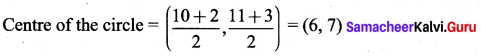Question 3.
Find the coordinates of the point which divides the line segment joining the points (3, 1) and (5, 13) internally in the ratio 3 : 5.
Solution: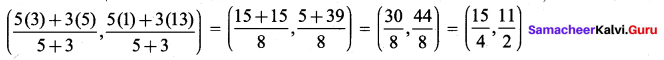Exercise 5.4

Question 1.
Using section formula, show that the points A(7, -5), B(9, -3) and C(13, 1) are collinear.
Solution: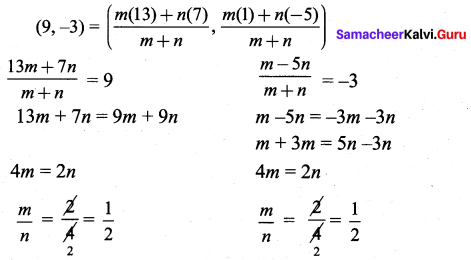Question 2.
A car travels at an uniform speed. At 2pm it is at a distance of 5 km at 6 pm it is at a distance of 120 km. Using section formula, find at what distance it will reach 2 mid night.
Solution: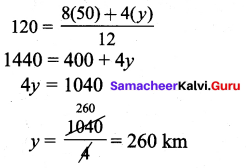Question 3.
Find the coordinates of the point which divides the line segment joining the point A(3, 7) and B(-11, -2) in the ratio 5 : 1.
Solution: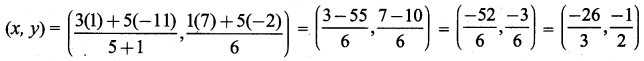Exercise 5.5

Question 1.
Find the centroid of the triangle whose vertices are (2, -5), (5, 11) and (9, 9)
Solution: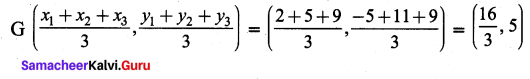Question 2.
If the centroid of a triangle is at (10, -1) and two of its vertices are (3, 2) and (5, -11). Find the third vertex of the triangle.
Solution: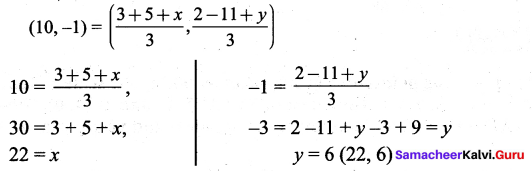Exercise 5.6

Multiple Choice Questions :

Question 1.
The point (-2, 7) lies is the quadrant
(1) I
(2) II
(3) III
(4) IV
Hint:
(-, +) lies in IInd quadrant
Solution:
(2) II

Question 2.
The point (x, 0) where x < 0 lies on
(1) OX
(2) OY
(3) OX’
(4) OY’
Hint:
(-, 0) lies on OX’
Solution:
(3) OX’

Question 3.
For a point A(a, b) lying in quadrant III.
(1) a > 0, b < 0
(2) a < 0, b < 0
(3) a > 0, b > 0
(4) a < 0, b > 0
Hint:
(-, -) lies in IIIrd quadrant
Solution:
(2) a < 0, b < 0

Question 4.
The diagonal of a square formed by the points (1, 0) (0, 1) and (-1, 0) is
(1) 2
(2) 4
(3) $$\sqrt{2}$$
(4) 8
Hint: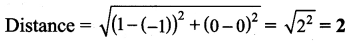Solution:
(1) 2Question 5.
The triangle obtained by joining the points A(-5, 0) B(5, 0) and C(0, 6) is
(1) an isosceles triangle
(2) right triangle
(3) scalene triangle
(4) an equilateral triangle
Hint:
Triangles having two sides equal are called isosceles.
Solution:
(a) an isosceles triangle

Text Book Activities

Activity 1.
Plot the following points on a graph sheet by taking the scale as 1cm = 1 unit. Find how far the points are from each other? A (1, 0) and D (4, 0). Find AD and also DA. Is AD = DA? You plot another set of points and verify your result.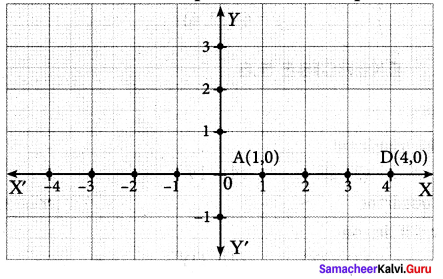Solution: